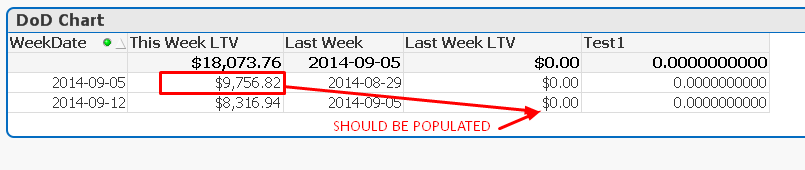# QlikView Creating Analytics

Discussion Board for collaboration related to Creating Analytics for QlikView.Not applicable

## Set Analysis for Dynamic Past Two Year Total

Hello,

I try to get dynamic sum of sale for previous two years. For example, when I select 2011, I would like to see the total sum of sale for Year 2010 and 2009, and when I select 2012, I would like to see the total sum of sale for Year 2011 and 2010. In the set analysis, which expression am I supposed to put for the field "Sale Year"?

Thank you so much!

8 RepliesMVP & Luminary

## Re: Set Analysis for Dynamic Past Two Year Total

Try: sum({<[Sale Year]={'>=\$(=only(Sale Year])-2)<\$(=only([Sale Year]))'}>} sale_amount)

Note: set analysis calculates one set for the entire chart, not a set per row. That means you can't use Sale Year as dimension. You have to select exactly one year.

talk is cheap, supply exceeds demandMVP

## Re: Set Analysis for Dynamic Past Two Year Total

HI

Try this

sum({<[Sale Year]={'>=\$(=max(Sale Year])-2)<\$(=max([Sale Year]))'}>} sale_amount)

Hope it helps

Valued Contributor II

## Re: Set Analysis for Dynamic Past Two Year Total

=sum({\$<year={"*"},year-={"\$(=Max(year))"}>}sale_amount)Not applicable

## Re: Set Analysis for Dynamic Past Two Year Total

So, what if I potentially have other date dimension, such as month or monthname. If I don't want the sum value to change even though I select a specific month/month name variables, should I use the following expression:

sum({<[Sale Year]={'>=\$(=only(Sale Year])-2)<\$(=only([Sale Year]))', [Sale Month]=, [Sale Month Name]=, [ Sale Date]=}>}?

Thanks!Not applicable

## Re: Set Analysis for Dynamic Past Two Year Total

So, what if I potentially have other date dimension, such as month or monthname. If I don't want the sum value to change even though I select a specific month/month name variables, should I use the following expression:

sum({<[Sale Year]={'>=\$(=only(Sale Year])-2)<\$(=only([Sale Year]))', [Sale Month]=, [Sale Month Name]=, [ Sale Date]=}>}?

Thanks!Not applicable

## Re: Set Analysis for Dynamic Past Two Year Total

So, what if I potentially have other date dimension, such as month or monthname. If I don't want the sum value to change even though I select a specific month/month name variables, should I use the following expression:

sum({<[Sale Year]={'>=\$(=only(Sale Year])-2)<\$(=only([Sale Year]))', [Sale Month]=, [Sale Month Name]=, [ Sale Date]=}>}?

My dimension in Chart is actually mm/yyyy, and I want ALL the months of a particular year show up the same total sale value for previous two year. Do you know what would be a good way to do it?

Thanks a lot!Not applicable

## Re: Set Analysis for Dynamic Past Two Year Total

So, what if I potentially have other date dimension, such as month or monthname. If I don't want the sum value to change even though I select a specific month/month name variables, should I use the following expression:

sum({<[Sale Year]={'>=\$(=only(Sale Year])-2)<\$(=only([Sale Year]))', [Sale Month]=, [Sale Month Name]=, [ Sale Date]=}>}?

My dimension in Chart is actually mm/yyyy, and I want ALL the months of a particular year show up the same total sale value for previous two year. Do you know what would be a good way to do it?

Thanks a lot!Not applicable

## Re: Set Analysis for Dynamic Past Two Year Total

I have a similar issue, where I have a dimension called Start Week, which is the first day of the week in YYYY-MM-DD.   I want to calculate based on each rows "Start Week", the previous weeks sales.  So that I can do a WoW delta.  I don't want to use the "Above" function because this would force the user to show two rows of data.

For example, if they selected one single start week selection, "2014-09-05"  then only one row should be displayed